# Chemical Formulas and Chemical Compounds Chapter 7 Chemical

• Slides: 36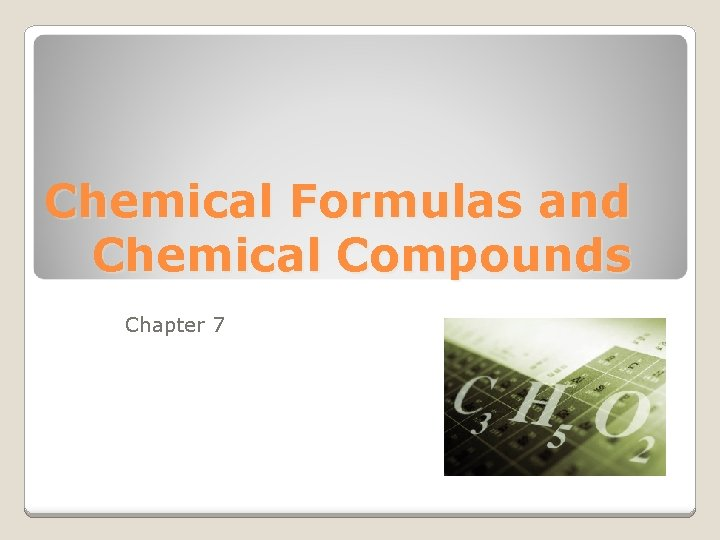Chemical Formulas and Chemical Compounds Chapter 7Chemical Names and Formulas Section 7 -1Definitions Chemical formula - Indicates the relative number of atoms of each kind in a chemical compound Molecular compound -The chemical formula reveals the number of atoms of each element contained in a single molecule of the compound Ionic compound - Consists of a lattice of positive and negative ions held together by mutual attraction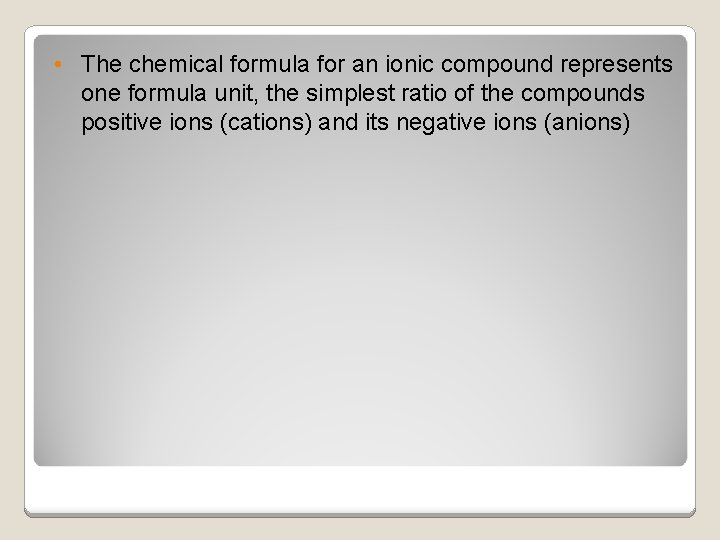• The chemical formula for an ionic compound represents one formula unit, the simplest ratio of the compounds positive ions (cations) and its negative ions (anions)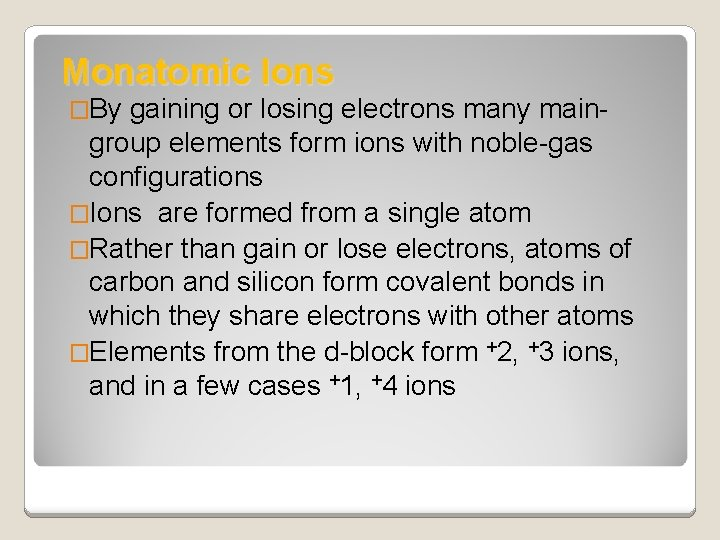Monatomic Ions �By gaining or losing electrons many main- group elements form ions with noble-gas configurations �Ions are formed from a single atom �Rather than gain or lose electrons, atoms of carbon and silicon form covalent bonds in which they share electrons with other atoms �Elements from the d-block form +2, +3 ions, and in a few cases +1, +4 ions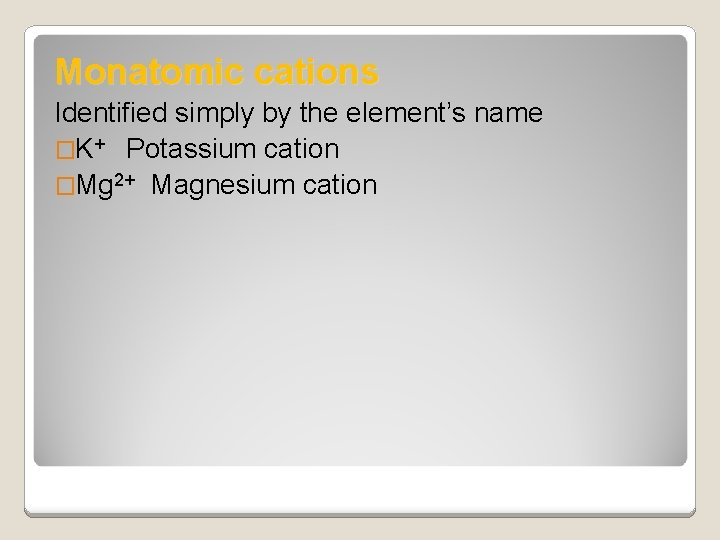Monatomic cations Identified simply by the element’s name �K+ Potassium cation �Mg 2+ Magnesium cation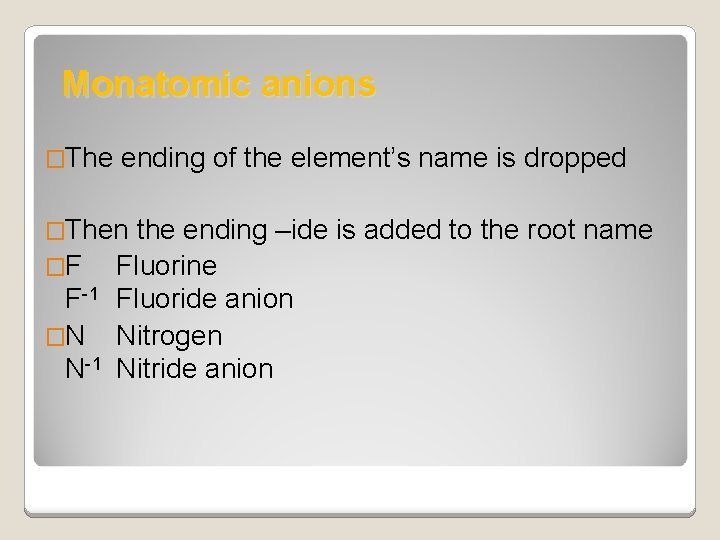Monatomic anions �The ending of the element’s name is dropped �Then the ending –ide is added to the root name �F F-1 �N N-1 Fluorine Fluoride anion Nitrogen Nitride anion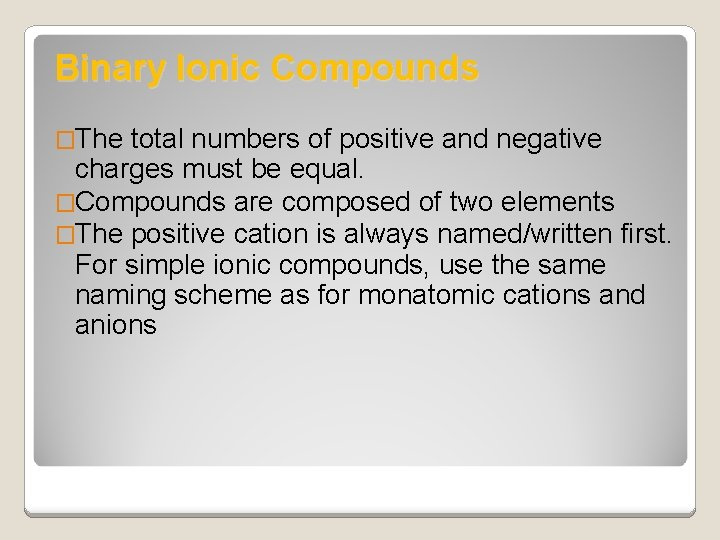Binary Ionic Compounds �The total numbers of positive and negative charges must be equal. �Compounds are composed of two elements �The positive cation is always named/written first. For simple ionic compounds, use the same naming scheme as for monatomic cations and anions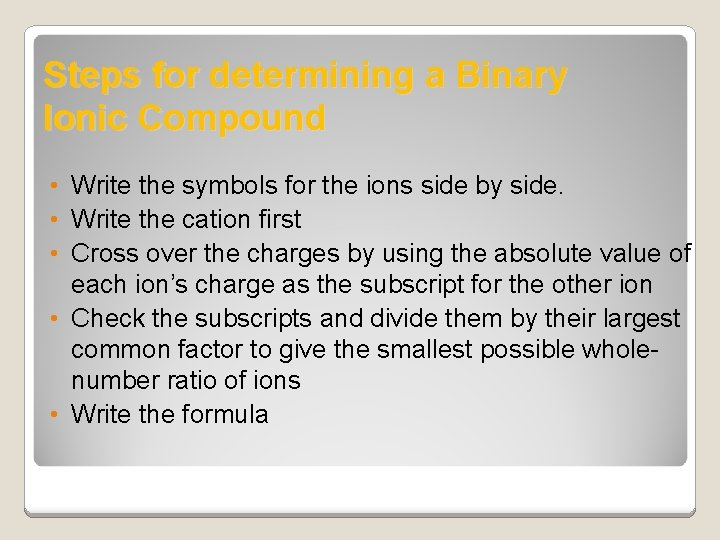Steps for determining a Binary Ionic Compound • Write the symbols for the ions side by side. • Write the cation first • Cross over the charges by using the absolute value of each ion’s charge as the subscript for the other ion • Check the subscripts and divide them by their largest common factor to give the smallest possible wholenumber ratio of ions • Write the formula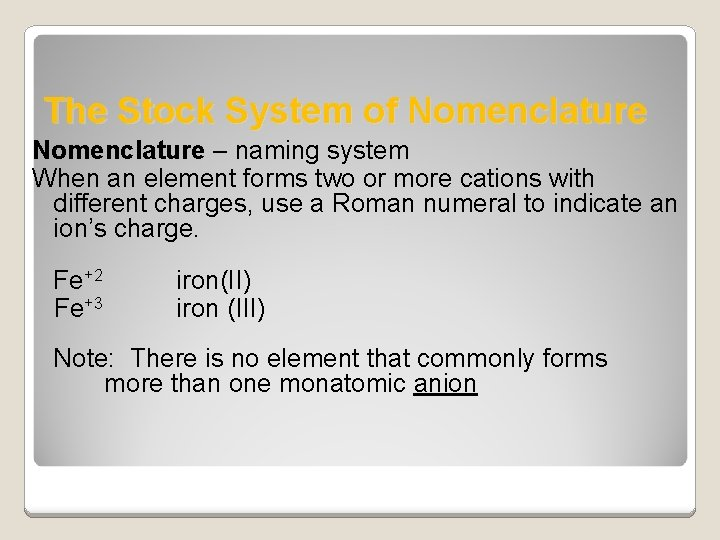The Stock System of Nomenclature – naming system When an element forms two or more cations with different charges, use a Roman numeral to indicate an ion’s charge. Fe+2 iron(II) Fe+3 iron (III) Note: There is no element that commonly forms more than one monatomic anionNaming According to the Stock System �Write the name of the cation �Indicate the charge with Roman numeral in parenthesis �Write the name of the anion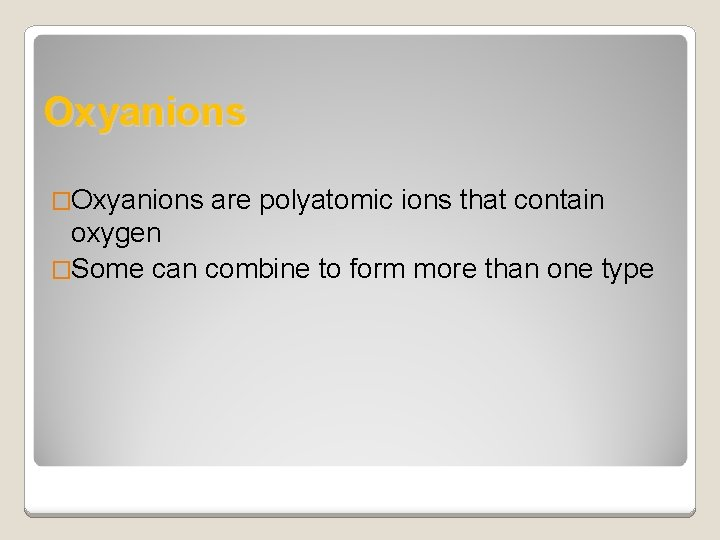Oxyanions �Oxyanions are polyatomic ions that contain oxygen �Some can combine to form more than one type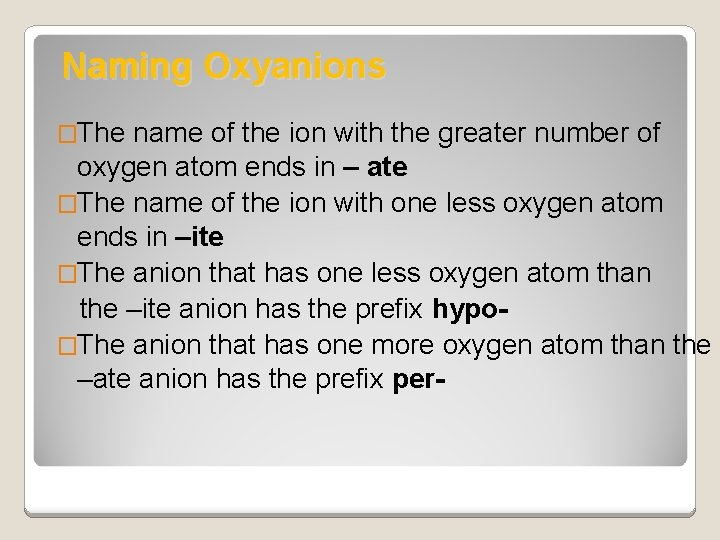Naming Oxyanions �The name of the ion with the greater number of oxygen atom ends in – ate �The name of the ion with one less oxygen atom ends in –ite �The anion that has one less oxygen atom than the –ite anion has the prefix hypo�The anion that has one more oxygen atom than the –ate anion has the prefix per-Examples of Oxyanions �Cl. O 2 - �Cl. O 3 - �Cl. O 4 - hypochlorite chlorate perchlorateNaming Binary Molecular Compounds Use prefixes to represent how many atoms needed. # of atoms needed 1 2 3 4 5 6 7 8 9 10 Prefix of Atom monoditritetrapentahexaheptaoctanonadeca-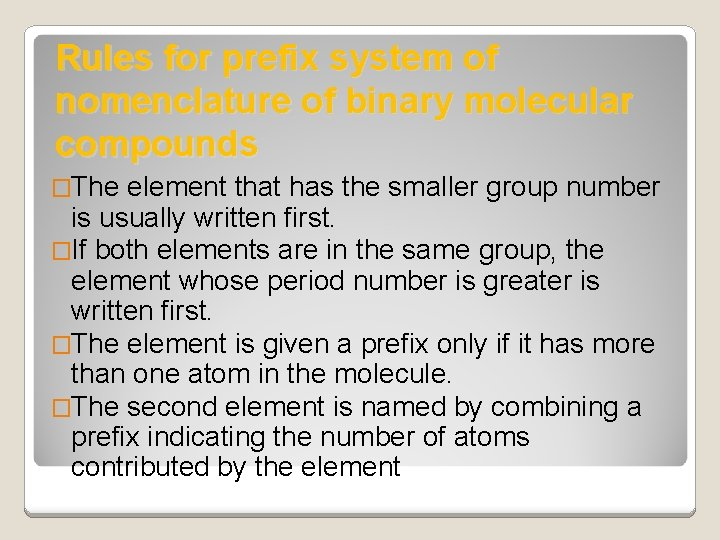Rules for prefix system of nomenclature of binary molecular compounds �The element that has the smaller group number is usually written first. �If both elements are in the same group, the element whose period number is greater is written first. �The element is given a prefix only if it has more than one atom in the molecule. �The second element is named by combining a prefix indicating the number of atoms contributed by the elementRules for prefix system of nomenclature of binary molecular compounds �Give the root of the name of the element and end in –ide (see note below) �The ‘o’ or ‘a’ at the end of a prefix is usually dropped when the word following the prefix begins with another vowel. For example, monoxide instead of mono-oxide pentoxide instead of penta-oxide The –ide indicates that the compound contains 2 elements or is binary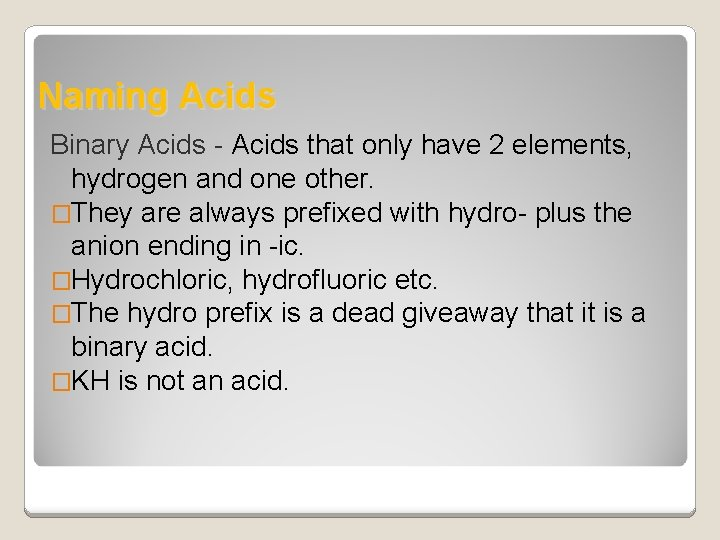Naming Acids Binary Acids - Acids that only have 2 elements, hydrogen and one other. �They are always prefixed with hydro- plus the anion ending in -ic. �Hydrochloric, hydrofluoric etc. �The hydro prefix is a dead giveaway that it is a binary acid. �KH is not an acid.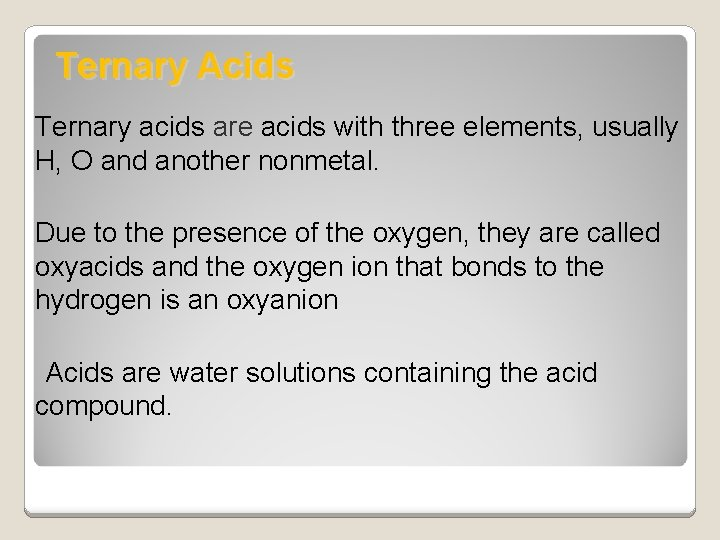Ternary Acids Ternary acids are acids with three elements, usually H, O and another nonmetal. Due to the presence of the oxygen, they are called oxyacids and the oxygen ion that bonds to the hydrogen is an oxyanion Acids are water solutions containing the acid compound.Naming Ternary Acids �The oxyanion the ending to that ends in -ate changes –ic acid the ends in –ite changes –ous acid.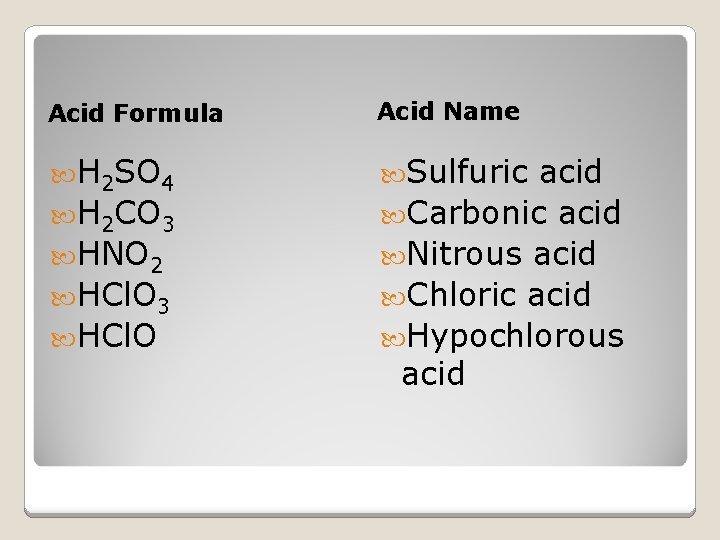Acid Formula Acid Name H 2 SO 4 Sulfuric H 2 CO 3 HNO 2 HCl. O 3 HCl. O acid Carbonic acid Nitrous acid Chloric acid Hypochlorous acid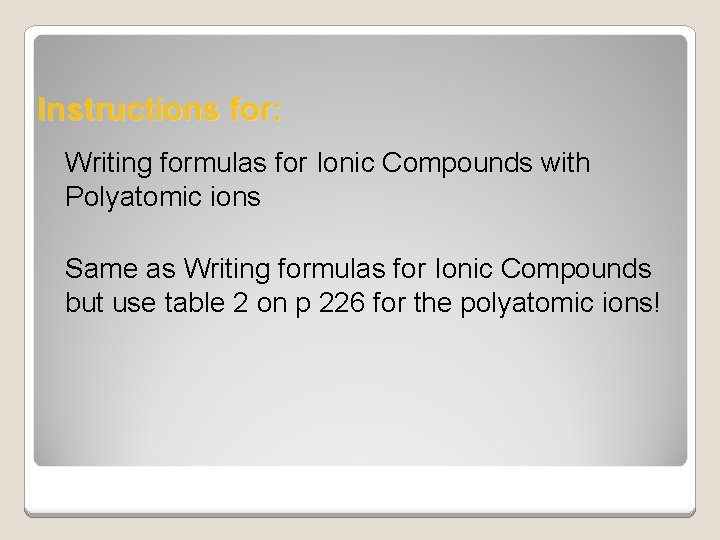Instructions for: Writing formulas for Ionic Compounds with Polyatomic ions Same as Writing formulas for Ionic Compounds but use table 2 on p 226 for the polyatomic ions!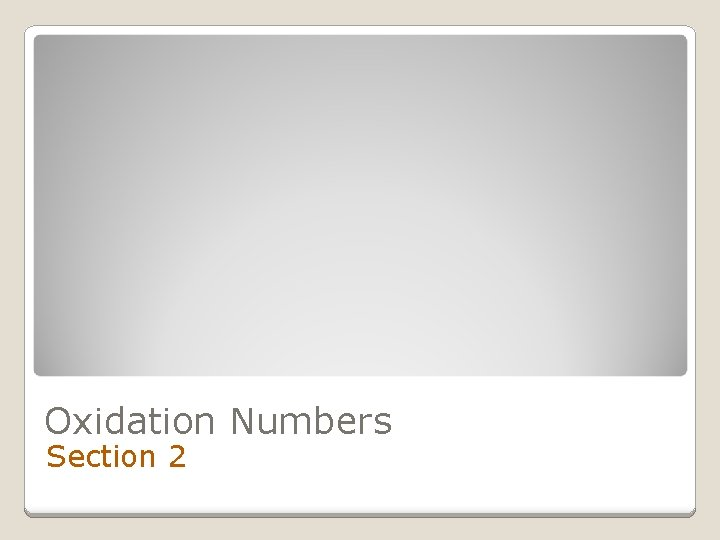Oxidation Numbers Section 2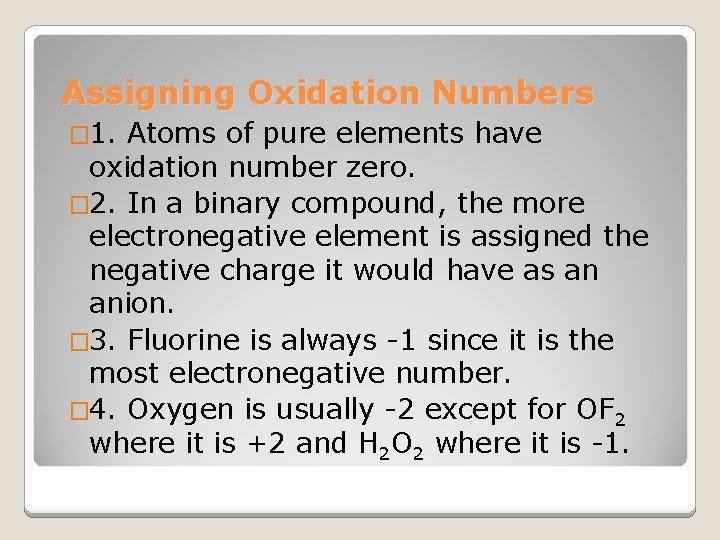Assigning Oxidation Numbers � 1. Atoms of pure elements have oxidation number zero. � 2. In a binary compound, the more electronegative element is assigned the negative charge it would have as an anion. � 3. Fluorine is always -1 since it is the most electronegative number. � 4. Oxygen is usually -2 except for OF 2 where it is +2 and H 2 O 2 where it is -1.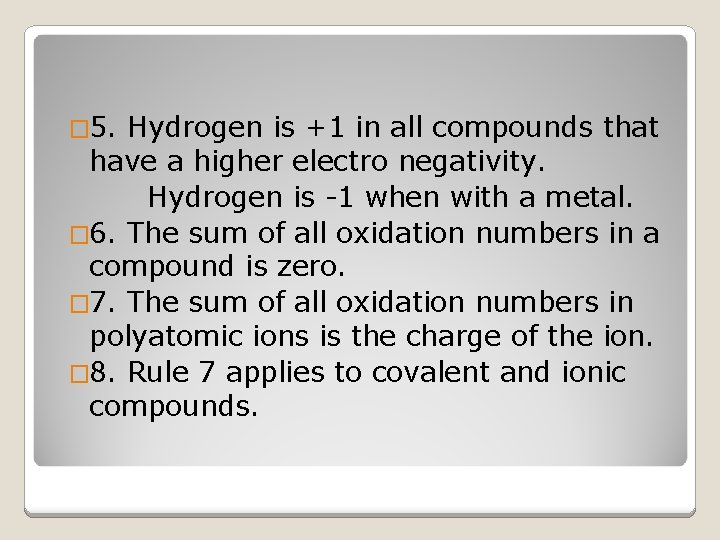� 5. Hydrogen is +1 in all compounds that have a higher electro negativity. Hydrogen is -1 when with a metal. � 6. The sum of all oxidation numbers in a compound is zero. � 7. The sum of all oxidation numbers in polyatomic ions is the charge of the ion. � 8. Rule 7 applies to covalent and ionic compounds.Examples H 2 SO 4 UF 6 Cl. O 3 -1 HNO 3 P 4 O 10 N 2 O 5 KH Oxidation Numbers S = +6 U = +6 Cl = +7 N = +5 P = +5 N = +5 H = -1Using Chemical Formulas Section 7. 3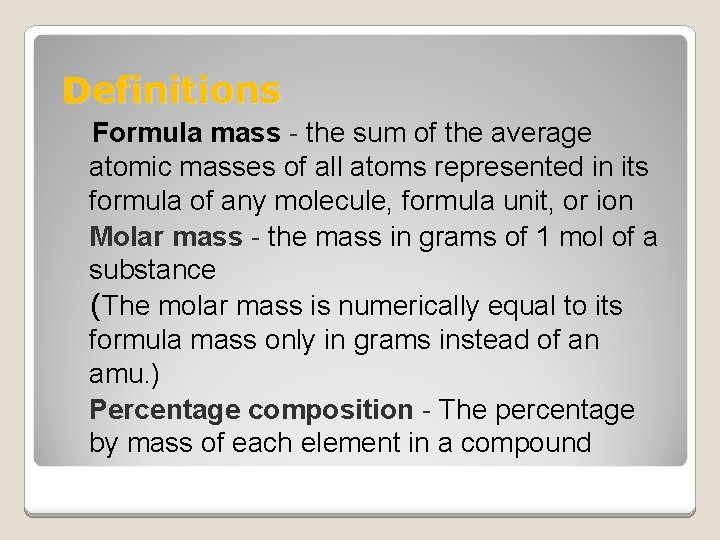Definitions Formula mass - the sum of the average atomic masses of all atoms represented in its formula of any molecule, formula unit, or ion Molar mass - the mass in grams of 1 mol of a substance (The molar mass is numerically equal to its formula mass only in grams instead of an amu. ) Percentage composition - The percentage by mass of each element in a compoundCalculate Percent Composition �Percent composition = composition of Cu 2 S. � 2 mol Cu x 63. 55 g Cu = 127. 10 g Cu 1 mol Cu � 1 mol S x 32. 07 g S = 32. 07 g S 1 mol S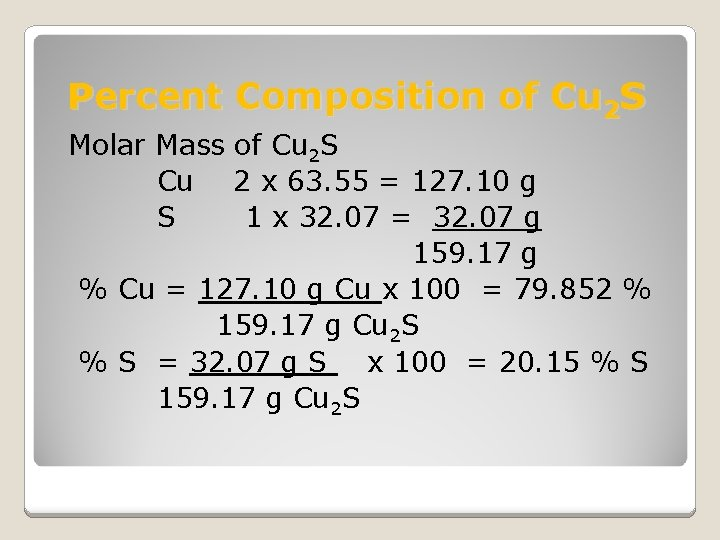Percent Composition of Cu 2 S Molar Mass of Cu 2 S Cu 2 x 63. 55 = 127. 10 g S 1 x 32. 07 = 32. 07 g 159. 17 g % Cu = 127. 10 g Cu x 100 = 79. 852 % 159. 17 g Cu 2 S % S = 32. 07 g S x 100 = 20. 15 % S 159. 17 g Cu 2 S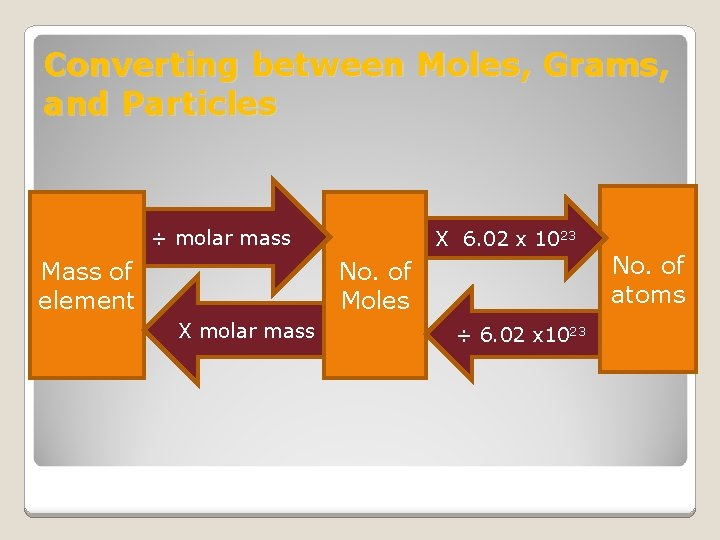Converting between Moles, Grams, and Particles ÷ molar mass Mass of element X 6. 02 x 1023 No. of Moles X molar mass ÷ 6. 02 x 1023 No. of atoms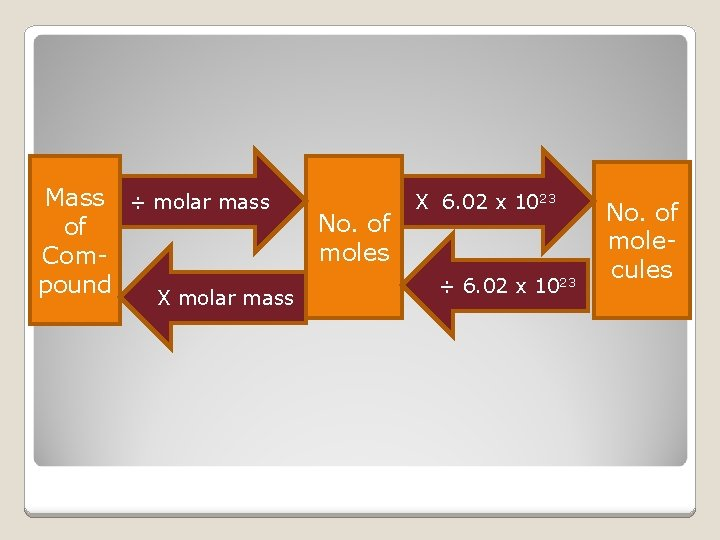Mass ÷ molar mass of Compound X molar mass No. of moles X 6. 02 x 1023 ÷ 6. 02 x 1023 No. of molecules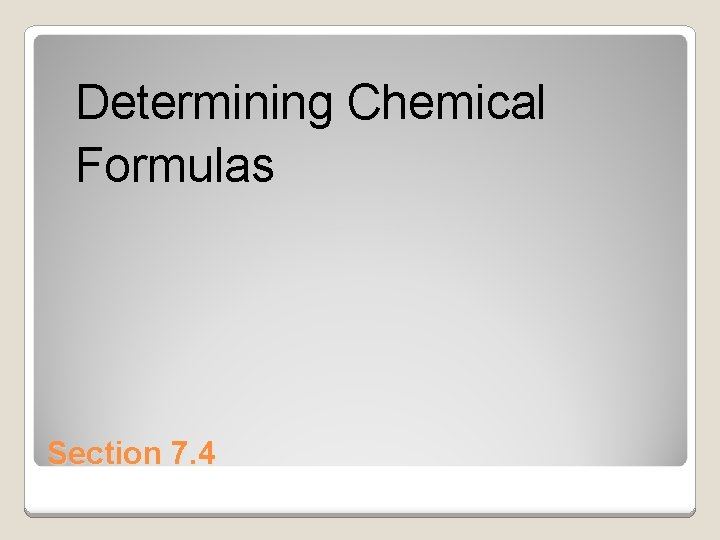Determining Chemical Formulas Section 7. 4Empirical formula �Consists of the symbols for the elements combined in a compound with subscripts showing the smallest whole-number mole ratio of the different atoms in the compound �Ionic compound, the formula unit is usually the compound’s empirical formula. �Molecular compound the empirical formula does not necessarily indicate the actual numbers of atoms present in each molecule